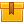# Introduction to Hydraulic Systems

None

## Description

This is the first lesson in the iKNOW® Industrial Hydraulic Power Library. This lesson identifies the basic components of an industrial hydraulic system and explains their functions. Formulas, including Pascal??s Law, are presented and their use in determining values in a hydraulic system is explained.

## Objectives

Visually identify the basic components of an industrial hydraulic system, Describe the function of the basic components of an industrial hydraulic system, Understand Pascal??s Law, Use Pascal??s Law to determine the pressure and flow at a given point in a simple hydraulic system and Using a diagram of a simple hydraulic system, describe the transmission of power through the system.

## ContentThis is the first lesson in the iKNOW™ Industrial Hydraulic Power
Library. This lesson identifies the basic components of an industrial
hydraulic system and explains their functions. Formulas, including
Pascal’s Law, are presented and their use in determining values in a
hydraulic system is explained.

•Introduction to Hydraulic Systems

0
0 Reviews